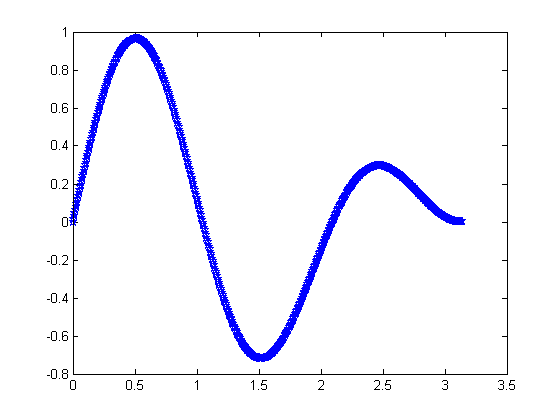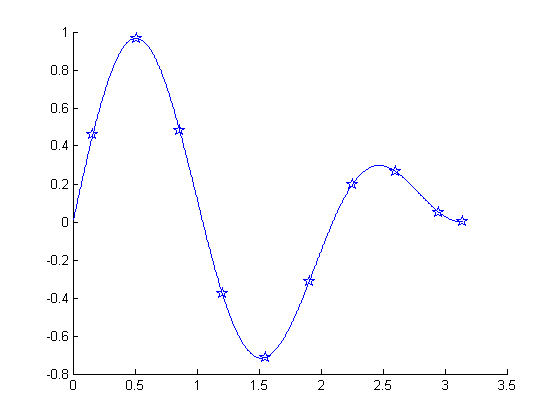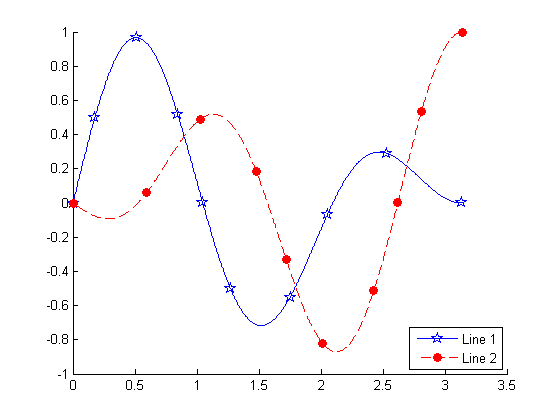# Line plots with fewer markers

Jiro's pick this week is line_fewer_markers by Massimo Ciacci.

"How can I set the intervals of markers in a plot?"

This is a question that I've seen at various times, both in MATLAB Answers and in MATLAB Newsgroup. The question is in regards to the fact that when MATLAB puts markers on a line plot, it puts them on every data point. For plots with many data points, it becomes visually crowded.

t  = 0:0.005:pi;
plot(t, sin(3*t).*cos(t/2), 'p-')line_fewer_markers allows you to specify the number of markers to show, regardless of how many data points are plotted.

clf;
line_fewer_markers(t, sin(3*t).*cos(t/2), 10, 'p-');It has some additional options, such as controlling how the spacing is calculated. This is useful especially when the line is curvey. In such cases, you may want to compute the marker spacing based on the distance on the curve, rather than based on the x distance.

clf;
line_fewer_markers(t, sin(3*t).*cos(t/2), 10, 'p-', 'Spacing', 'curve');And finally, the function can return a handle that can be used specifically for legend, so that it displays the correct marker and line style.

clf;
h1 = line_fewer_markers(t, sin(3*t).*cos(t/2), 10, 'p-', 'Spacing', 'curve');
h2 = line_fewer_markers(t, -sin(t/2).*cos(3*t), 10, 'o--r', 'Spacing', 'curve', ...
'MarkerSize', 6, 'MarkerFaceColor', 'r');
legend([h1, h2], 'Line 1', 'Line 2', 'Location', 'SouthEast');Massimo's function is well-documented, and he also includes a few example scripts to show the different use cases.